Next: Interpretation Up: Two narrow slits Previous: Two narrow slits

### Analysis

We now consider the simplest case which exhibits interference, two equivalent, narrow slits (Figure 3). Of course, you can get the two-slit pattern by substituting N=2 into the formula we shall eventually derive for N slits. But, deriving the two slit result as a special case first allows us to illustrate several useful results and techniques for complex arithmetic that you will have to master, in particular computing the amplitude of the complex number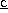with the formula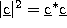. This section appears very mathematical because we show all of the steps. As you learn to do these steps, they will become simpler and simpler for you.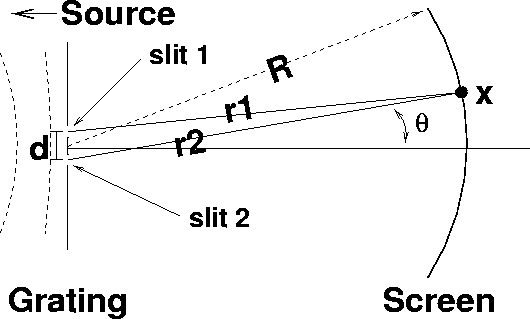Figure 3: Double-slit interference experiment

According to our main result, Eq. 8, the intensity at a point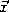on the screen for just two slits is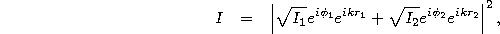where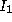and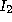,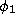and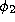, and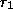and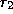, respectively, are (a) the intensities which each slit (if alone) would make at point, (b) the phases of the waves emerging from each slit, and (c) the distance from each slit to the point.

Now, because we assume the two slits to be equivalent, they must let the same intensity of waves through, and so the intensities which each separately would create on the screen are very nearly the same, so that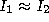. (There will be a slit difference in the distance from each slit to the pointon the screen, but so long as the screen is much further away than the separation of the slits, R>>a, this difference is negligible.) For simplicity, let us call this intensity which either slit alone creates on the screen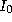.

Next, because the waves coming from behind the diffraction grating arrive at both slits in phase (Figure 3), the phases of the waves coming out of the slits will be equal,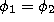. For simplicity, let us call this phase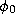. As we shall see in a moment, the fact that these phases are equal means that they cancel from the final result. Note that this is only true if the waves coming from behind the grating arrive at the slits with equal phases!

Putting this all together we have,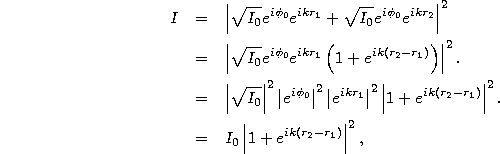where, again, we have used the facts that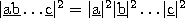and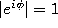for any complex phase factor. Note that, as we had mentioned, in the end, the phases indeed cancel out. Also note that the entire result now depends only on the difference in lengths between the paths from the observation point to each slit,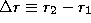.

The entire computation of I now boils down to finding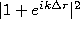. A very useful way to find the square-magnitude of a complex numberis to use the following fact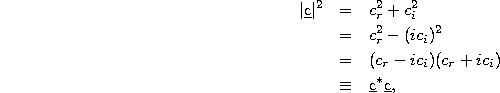where, for any complex number, we define the complex conjugate as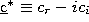. Mathematically, taking the complex conjugate simply amounts to finding all occurrences of i and substituting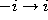.

Using the procedure of Eq. 12, we now find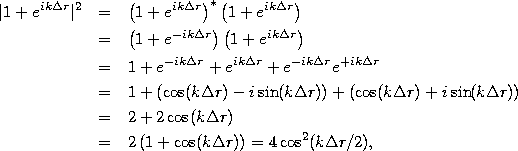where on the last line we find a somewhat simpler form by using the trig identity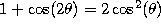. Combining this with Eq. 10, we have the final result for two-slits,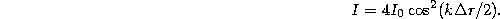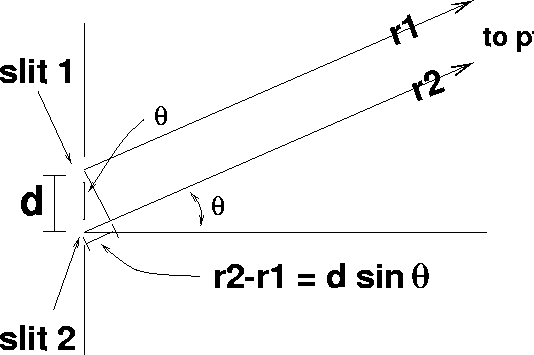Figure 4: Double-slit interference experiment: enlarged view

All that remains is for us to learn to compute the path difference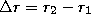. Figure 4 shows that the path difference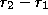corresponds very closely to the opposite side of a right triangle of angleand hypotenuse d. Accordingly, our final result is Eq. 13 with the definition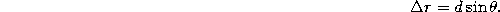Next: Interpretation Up: Two narrow slits Previous: Two narrow slits

Tomas Arias
Thu Sep 13 15:26:14 EDT 2001